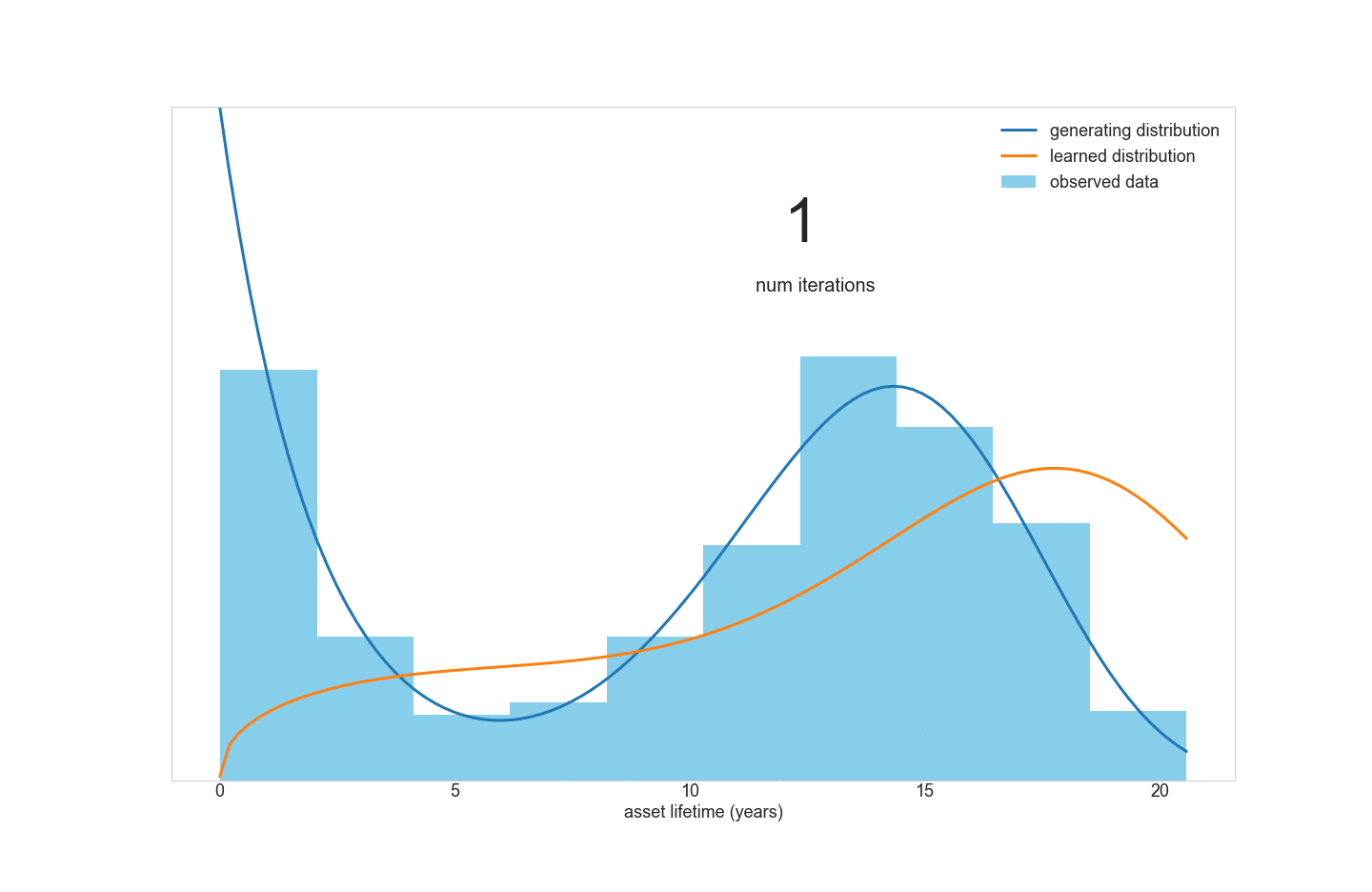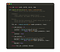# How to use Jax to streamline machine learning optimization

## Speed up your optimization processes and reduce development time with this open source Python library.

TL; DR: Without reliable optimization methods, training ML models is infeasible or too slow to be practically useful. Many of the most powerful optimization methods require calculating loss function derivatives in order to efficiently search the space of possible parameter values. However, computing derivatives of complicated functions is tedious, time-consuming, and prone to human error. Jax is an open-source Python library that provides an automated method for computing derivatives, reducing development cycle time and improving the readability of code.

# Optimization for ML: an overview

Many machine learning problems can be boiled down to the following steps:

• Specify a model with parameters θ that takes in input data and returns a predicted value or set of values.
• Specify a loss function that captures how dissimilar the predictions of a model are compared to observed “ground truth” data (of course, this is highly problem-dependent).
• Find the set of parameters θ that minimize the loss function using an optimization routine.

Consider the case of linear regression with two independent variables x1 and x2. The model specification is:

Here, the β values represent the parameters that need to be optimized (taking the place of θ in our more general description above) , ε is an error term that represents uncertainty, and y is the “true” value that we’re trying to predict. The canonical loss function for linear regression is the sum of squared errors:

This applies across all of our n data points. Therefore, we’d like to pick the β values such that this value is minimized. In the specific case of linear regression, we can actually solve for the β values analytically using linear algebra. However, in most cases, finding an analytic solution in this way is not possible. Therefore, we turn to optimization methods which allow us to iteratively search for the best parameter values that minimize the loss function for whatever problem we’re trying to solve.

# Why do we need derivatives?

The space of possible parameter values is usually infinite, so we need a clever way to search for the optimal parameters. Consider the analogy of a blind person lost in the hills who wants to get back to the valley where they parked their car. (Imagine the hills are completely smooth and there are no obstacles to contend with). At each point, they can sense the slope of the hill, and so they proceed by walking downhill, re-calibrating every so often to figure out the direction of the steepest downwards descent and then walking in that direction.

In this analogy, the person’s elevation corresponds to the loss function they want to minimize, and the x and y coordinates of the direction they walk in represent the two parameters of this “model”. This is the essence of many iterative derivative-based optimization methods: by understanding the topography of the region in parameter space, one can determine the direction that most quickly decreases the loss function and hopefully reach the global minimum most quickly.

# How can Jax help?

For many machine learning models, calculating derivatives of loss functions by hand is downright painful. Online tools such Wolfram Alpha can help accelerate this process, but they still require the user to write Python code to encode the derivative correctly. This isn’t so hard for scalar data inputs, but in practice many machine learning models take vectors or matrices as input, and one incorrectly applied dot product or summation can lead to an incorrect derivative that will crash the optimization routine. If only there was a way to get the computer to do all of this hard work for you….

Enter Jax, an open-source library created by Google that can automatically compute derivatives of native Python and NumPy¹ functions. This means that as a user, you can write numerical functions in Python and use Jax to automatically compute derivatives (including higher-order derivatives!) and use them as input to optimization routines.

Under the hood, Jax makes use of the technique of autodifferentation, a topic that falls squarely outside the scope of this post (this blog post gives a helpful introduction, though). The good news is, you don’t have to understand anything about how autodiff works² in practice to use Jax — you just need to know the basics of how to set up an optimization problem!

Let’s walk through a real-life example of how Jax can be used to simplify the implementation of a machine learning model.

# Using Jax for fitting Weibull mixture models to data

One of Tagup’s main algorithmic approaches for improving the decision making of asset managers is our time-to-event (TTE) modeling capabilities. Our TTE models use the unified data history of an asset to predict time until certain events occur such as machine failures, preemptive removals, or maintenance actions (for the remainder of this post, we will discuss the specific case of predicting time until failure, commonly known as survival modeling).

Our convex latent variable (CLV) model works by first fitting a parametric distribution to observed asset lifetimes and then using other available data (nameplate data, sensor readings, weather, etc.) to scale that distribution which we refer to as our base hazard. In future posts, we will discuss our approach to time-to-event modeling in detail (this also involves interesting optimization techniques!), but for the purpose of this post, we will focus on the simpler sub-problem of fitting a distribution to observed machine lifetimes.

One of the most common distributions used for modeling machine lifetimes is the Weibull distribution, which is a very flexible distribution that is governed by only two parameters. (See our previous post for a brief introduction to survival modeling and an explanation of fitting a Weibull model to data.)

However, consider a fleet of assets that contains two distinct sub-populations with different means and variances of lifetimes. A simple Weibull model won’t be able to fit this data very well, because the data is really a mixture of multiple distributions. This inspired us to create a tool for fitting Weibull mixture models to data.A Jax-powered optimization routine learning the optimal Weibull mixture model parameters from synthetically generated asset lifetimes. By looping through the predicted distribution at each iteration, we can see how the routine “learns” the true distribution from which the data was generated.

A quick literature review turned up this research paper for doing just that. However, the optimization routine outlined in the paper didn’t look appealing to implement in Python:

How could we avoid the painful process of turning the derivatives into code? The answer, of course, is to use Jax:An example of computing derivatives of an arbitrary function using Jax.

Let’s briefly run through what’s going on in this function, the purpose of which is to compute the hazard³ as a function of model parameters and time since install as well as derivatives of this function if requested.

1. Since compute_hazard is a function of multiple parameters, the derivatives required for optimization are matrices known as the Jacobian (first derivative) and Hessian (second derivative), hence the variable names.
2. The Jax function grad is used to compute the first derivative of the hazard function, while vmap automatically vectorizes the gradient computation across the inputs (see docs and examples here).
3. Lastly, jacfwd and jacrev are used in succession to compute the Hessian matrix if requested by the user.

Wow. In a few simple lines of code, we were able to compute the first and second derivative of the hazard function. Now, all we have to do is pass functions for computing loss and its derivatives to an optimization routine (the specifics of which will be detailed in a future post), and voila, we’re able to find the optimal parameters!

# Conclusion

Optimization is an essential part of making any machine learning problem feasible. Derivative-based optimization methods are by far the most common and reliable approaches used, but require that derivatives be worked out by hand which is often quite tedious and prone to error.

Jax is an open-source library that seamlessly integrates with Python and uses autodifferentiation to efficiently compute derivatives of complex functions, obviating the need to calculate them manually. It generalizes to many classes of problems common in machine learning and is a very useful tool for data scientists who write custom optimization routines.

In a future post, we’ll talk more about some considerations and limitations of Jax which will hopefully help you avoid some of the pitfalls we ran into when getting familiar with the library.

¹ NumPy is a widely used Python package that provides efficient implementations for numerical computations of the type often required by machine learning models.

² Jax does have some important limitations that users have to be aware of, though — these will be described in a future post.

³ In the context of survival modeling, the hazard function represents the probability that an asset fails in period t+1 given that it has lived up until time t. Hazard is convenient to work with for two reasons: the likelihood function that we seek to optimize is a function of hazard; and all statistics of interest (failure probability, expected RUL) can be derived from hazard.

## When Machines Learn

Sharing research and insight into applying machine learning to industrial asset management.

## When Machines Learn

A blog to share research and work in applying machine learning in heavy industry. Focus includes asset management and process optimization.

Written by

## Sam McCormick

Data Scientist at Tagup Inc., specializing in survival modeling for industrial equipment.## When Machines Learn

A blog to share research and work in applying machine learning in heavy industry. Focus includes asset management and process optimization.